## Cramer's Rule

Consider the general 2 by 2 linear system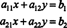Multiplying the first equation by a 22, the second by − a 12, and adding the results eliminates y and permits evaluation of x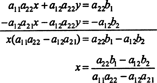assuming that a 11 a 22a 12 a 21 ≠ 0. Similarly, multiplying the first equation by − a 21, the second by a 11, and adding the results eliminates x and determines yagain assuming that a 11 a 22a 12 a 21 ≠ 0. These expressions for x and y can be written in terms of determinants as follows: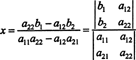andIf the original system is written in matrix form,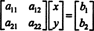then the denominators in the above expressions for the unknowns x and y are both equal to the determinant of the coefficient matrix. Furthermore, the numerator in the expression for the first unknown, x, is equal to the determinant of the matrix that results when the first column of the coefficient matrix is replaced by the column of constants, and the numerator in the expression for the second unknown, y, is equal to the determinant of the matrix that results when the second column of the coefficient matrix is replaced by the column of constants. This is Cramer's Rule for a 2 by 2 linear system.

Extending the pattern to a 3 by 3 linear system,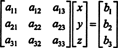Cramer's Rule says that if the determinant of the coefficient matrix is nonzero, then expressions for the unknowns x, y, and z take on the following form: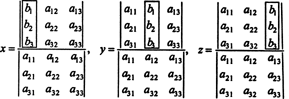The general form of Cramer's Rule reads as follows: A system of n linear equations in n unknowns, written in matrix form A x = b aswill have a unique solution if det A ≠ 0, and in this case, the value of the unknown x j is given by the expressionwhere A j is the matrix that results when column j of the coefficient matrix A is replaced by the column matrix b.

Theorem F. A square system A x = b will have a unique solution for every column matrix b if and only if det A ≠ 0.

Theorem G. A homogeneous square system A x = 0 will have only the trivial solution x = 0 if and only if det A ≠ 0.

Although Cramer's Rule is of theoretical importance because it gives a formula for the unknowns, it is generally not an efficient solution method, especially for large systems. Gaussian elimination is still the method of choice. However, Cramer's Rule can be useful when, for example, the value of only one unknown is needed.

Example 1: Use Cramer's Rule to find the value of y given that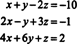Since this linear system is equivalent to the matrix equationCramer's Rule implies that the second unknown, y, is given by the expressionassuming that the denominator—the determinant of the coefficient matrix—is not zero. Row‐reduction, followed by Laplace expansion along the first column, evaluates these determinants:With these calculations, (*) implies